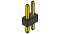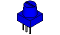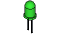NCIR08
 .:Twisting:..:Potentiometers:.(NEDX) .:Netduino Experimentation Kit:. (NEDX)

What We're Doing
Along with the digital pins, the Netduino also has 6 pins which can be used for analog input. These inputs take a voltage (from 0 to 3.3 volts) and convert it to a digital number between 0 (0 volts) and 1023 (3.3 volts) (10 bits of resolution). A very useful device that exploits these inputs is a potentiometer (also called a variable resistor). When it is connected with 3.3 volts across its outer pins the middle pin will read some value between 0 and 3.3 volts dependent on the angle to which it is turned (ie. 1.67 volts in the middle). We can then use the returned values as a variable in our program.

The Circuit
The PartsNCIR-08 Breadboard Sheet x12 Pin Headerx4Potentiometer10k ohm x1WireGreen LEDx1560 Ohm ResistorGreen-Blue-Brownx1SchematicResources
http://nedx.org/NBLS08Code (no need to type everything in just)
Download the Code from ( http://nedx.org/CODE08 )
(and then copy the text and paste it into an empty Netduino Sketch)

//Libraries that our program uses
using System;                               //use base classes
using Microsoft.SPOT;                       //use smart personal objects technology classes
using Microsoft.SPOT.Hardware;              //use hardware related SPOT classes
using SecretLabs.NETMF.Hardware;            //use Secret Labs hardware framework
using SecretLabs.NETMF.Hardware.Netduino;   //use the Netduino specific classes

namespace NCIR08                    /// Define the namespace we are in ///
{
public class Program            /// Define any variables used in the program and subrout
//ines after Main()///
{
static OutputPort led = new OutputPort(Pins.GPIO_PIN_D13, false);       //defines pi
//n 13 as connected to our LED
static AnalogInput potentiometer = new AnalogInput(Pins.GPIO_PIN_A0);   //defines ou
//r potentiometer as being connected to analog pin 0
static int sensorValue = 0;                                             //variable t
//o store our sensor value

public static void Main()   /// The Main loop (run at power up) ///
{
while (true)            /// Do Forever ///
{
sensorValue = potentiometer.Read();   // Read the potentiometer's value (ret
//urns a value between 0 and 1023)
led.Write(true);                      // Turns the LED on
Thread.Sleep(sensorValue);            // Waits sensorValue milliseconds
led.Write(false);                     // Turns the LED off
Thread.Sleep(sensorValue);            // Waits sensorValue milliseconds
}                       /// Close Forever Loop ///
}                           /// Close the Main() Loop ///
}                               /// Close the Program Loop ///
}                                   /// Close the Namespace Loop ///

Not Working? (3 things to try)

This is most likely due to a slightly dodgy connection with the potentiometer's pins. This can usually be conquered by taping the potentiometer down.

Not Working
Make sure you haven't accidentally connected the potentiometer's wiper to digital pin 2 rather than analog pin 2. (the row of pins beneath the power pins)

Still Backward
You can try operating the nciruit upside down. Sometimes this helps.

Making it Better?

Threshold switching:
Sometimes you will want to switch an output when a value exceeds a certain threshold. To do this with a potentiometer change the code to after while (true) to:

int threshold = 512;
if (sensorValue > threshold)
{
led.Write(true); // Turn the LED on
}
else
{
led.Write(false); // Turn the LED off
}

This will cause the LED to turn on when the value is above 512 (about halfway), you can adjust the sensitivity by changing the threshold value.

Let's control the brightness of an LED directly from the potentiometer. To do this we need to first change the pin the LED is connected to. Move the wire from pin 13 to pin 9

Then change the output pin declaration from:
static OutputPort led = new OutputPort(Pins.GPIO_PIN_D13, false);
|
static PWM led = new PWM(Pins.GPIO_PIN_D9);

Then change the code after while (true) to: